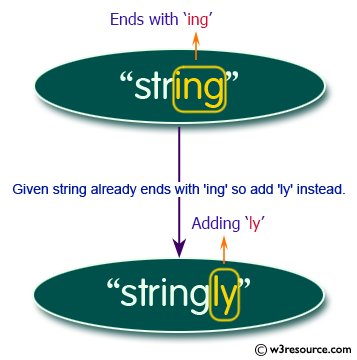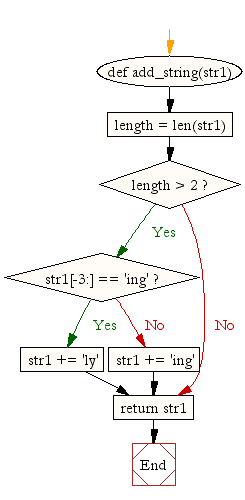﻿ Python: Add 'ing' at the end of a given string (length should be at least 3). If the given string already ends with 'ing' then add 'ly' instead. If the string length of the given string is less than 3, leave it unchanged - w3resource# Python: Add 'ing' at the end of a given string (length should be at least 3). If the given string already ends with 'ing' then add 'ly' instead. If the string length of the given string is less than 3, leave it unchanged

## Python String: Exercise-6 with Solution

Write a Python program to add 'ing' at the end of a given string (length should be at least 3). If the given string already ends with 'ing' then add 'ly' instead. If the string length of the given string is less than 3, leave it unchanged.Sample Solution:-

Python Code:

``````def add_string(str1):
length = len(str1)

if length > 2:
if str1[-3:] == 'ing':
str1 += 'ly'
else:
str1 += 'ing'

return str1
```
```

Sample Output:

```ab
abcing
stringly
```

Flowchart:## Visualize Python code execution:

The following tool visualize what the computer is doing step-by-step as it executes the said program:

Python Code Editor:

Have another way to solve this solution? Contribute your code (and comments) through Disqus.

What is the difficulty level of this exercise?

Test your Programming skills with w3resource's quiz.

﻿

## Python: Tips of the Day

Check if a given key already exists in a dictionary:

In is the intended way to test for the existence of a key in a dict.

```d = {"key1": 10, "key2": 23}

if "key1" in d:
print("this will execute")

if "nonexistent key" in d:
print("this will not")
```

If you wanted a default, you can always use dict.get():

```d = dict()

for i in range(100):
key = i % 10
d[key] = d.get(key, 0) + 1
```

and if you wanted to always ensure a default value for any key you can either use dict.setdefault() repeatedly or defaultdict from the collections module, like so:

```from collections import defaultdict

d = defaultdict(int)

for i in range(100):
d[i % 10] += 1
```

but in general, the in keyword is the best way to do it.

Ref: https://bit.ly/2XPMRyz